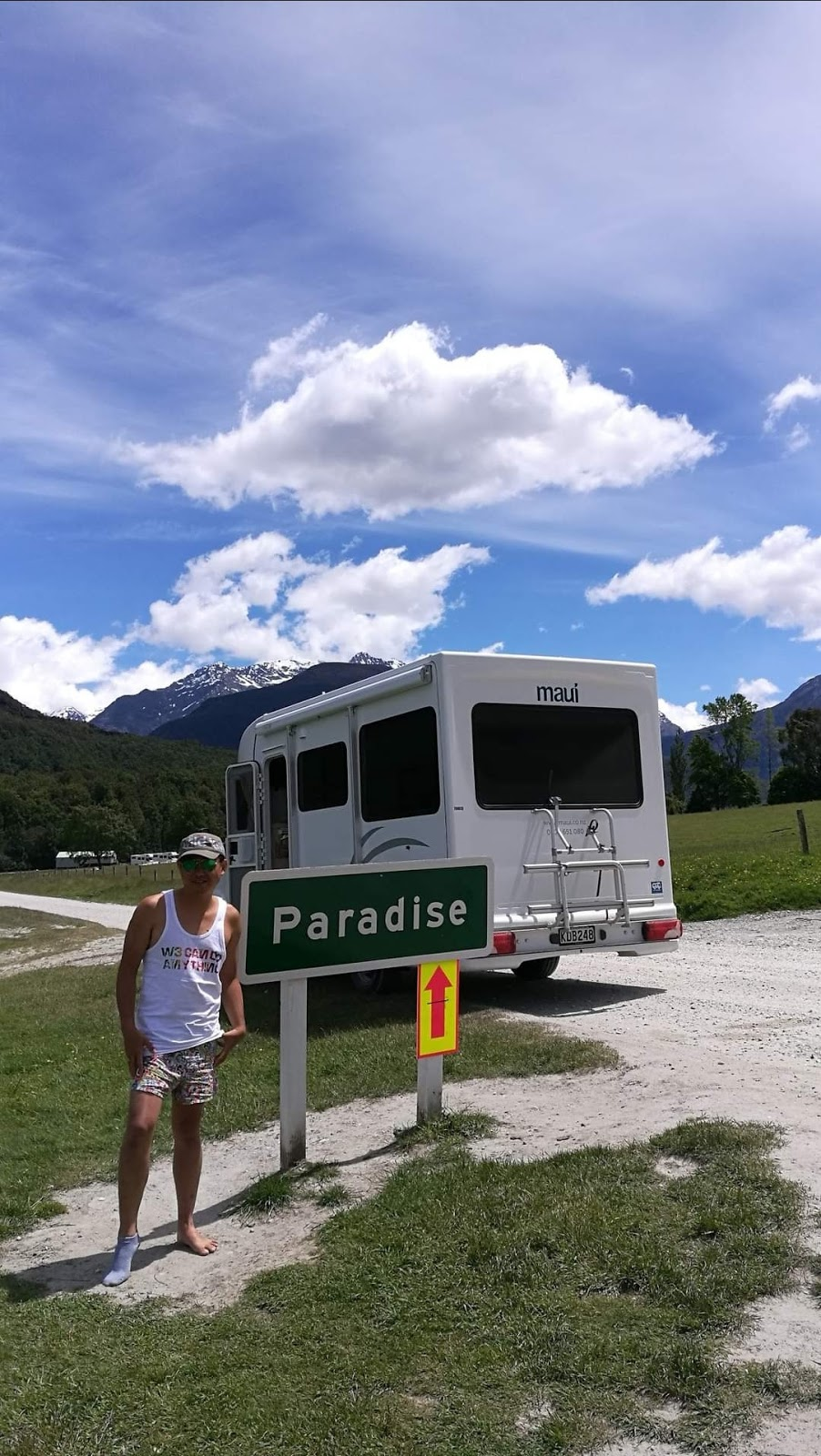## 星期二, 九月 22, 2020

### 天堂之路，不需行李摄于 纽西兰南部 2016

^^^^^^^^^^^^^^^^^^^^^^^^^^^^^^^^^^^^^^^^^^^^^

## 星期五, 九月 18, 2020

### 没有结果的努力，是毫无意义的吗?

^^^^^^^^^^^^^^^^^^^^^^^^^^^^^^^^^^^^^^^^^^^^^

## 星期三, 九月 09, 2020

### 我谈 《 平凡的荣耀》

有位女孩私讯了我，要我看这部然后写影评。其实这类题材不吸引我，加上全部的主角我也不怎么喜欢。要我看，真的难上加难。

《平凡的荣耀》的含义，就是在平凡中找到属于自己的路，创造属于自己的荣耀。^^^^^^^^^^^^^^^^^^^^^^^^^^^^^^^^^^^^^^^^^^^^^^^^^^^^^^^^^^^^^^^^^^^^^^^^^^^^^^^^^^^^^^^^“一个人怎么能决定自己的卖点呢？能不能成为卖点，不是应该看别人需不需要吗？ 一个人再怎么努力，如果不能满足对方的需求的话，那别人又凭什么去购买它，所以这个问题本身就是不成立的。” 孙弈秋

《平凡的荣耀》从未避讳职场的艰难与痛苦，却仍给予观众信心。从而去引导更多为生活所困的普通人以正确积极的姿态面对世界，让他们在共鸣中获得力量与勇气。

^^^^^^^^^^^^^^^^^^^^^^^^^^^^^^^^^^^^^^^^^^^^^^^^^^^^^^^^^^^^^^^^^^^^^^^^^^^^^^^^^^^^^^^^

"未到终局，焉知生死? " 这句已经从主角口中出现了多次。这就是经典。

## Welcome to My Movie World